## Line filter for opencamlib

When generating toolpaths with drop-cutter ("axial tool-projection", if you like fancy words) the path ends up being composed of lots of short linear segments. It makes sense to filter this list of points and take out any middle points that lie on a straight line between neighboring points. Here's a first attempt at such a line-filter.

The lower points is the raw output from drop cutter, while the filtered points are offset vertically for clarity. There are 6611 CL-points in the raw data, but only 401 CL-points after filtering.

The next step is to come up with an arc-filter which detects circular arcs in the list of CL-points. All CNC-machines have G2/3 circular arc motion in the principal planes (xy, xz, yz). Then the number of moves will go even further down from 401 points.

This is just something to live with, having chosen the triangulated model approach to CAM. First we have pure and exact shapes in CAD which get tessellated into zillions of tiny triangles, from these we generate a sampled toolpath consisting of lots and lots of points, and finally we filter to get back those "pure" "exact" and "nice" line-segments and arcs.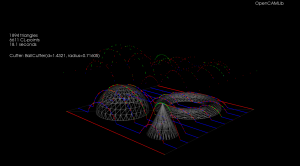The relevant part of the code looks like this. It might be a bit prettier to use the remove_if STL algorithm? Also, there's no check that p1 actually lies between p0 and p2.

```1 2 3 4 5 6 7 8 9 10 11 12 13 14 15 16 17 typedef std::list<clpoint>::iterator cl_itr; cl_itr p0 = clpoints.begin(); cl_itr p1 = clpoints.begin(); p1++; cl_itr p2 = p1; p2++; for( ; p2 != clpoints.end(); ) { Point p = p1->closestPoint(*p0, *p2); if ( (p- *p1).norm() < tol ) { // p1 is to be removed p1 = clpoints.erase(p1); p2++; } else { p0++; p1++; p2++; } }```

full code here.

## Matrix determinant with Boost::uBLAS

Boost uBLAS provides BLAS functionality, but doesn't have a function for computing the determinant of a matrix. Googling for this turns up a few code snippets, but it's best to document this completely here now since I got it to work, and it will be useful for opencamlib sooner or later.

```1 2 3 4 5 6 7 8 9 10 11 12 13 14 15 16 17 18 19 20 21 22 23 24 25 26 27 28 29 30 31 32 33 34 35 36 37 38 39 40 41 42 #include <boost /numeric/ublas/matrix.hpp> #include </boost><boost /numeric/ublas/io.hpp> #include </boost><boost /numeric/ublas/lu.hpp>   namespace bnu = boost::numeric::ublas;   int determinant_sign(const bnu::permutation_matrix<std ::size_t>& pm) { int pm_sign=1; std::size_t size = pm.size(); for (std::size_t i = 0; i < size; ++i) if (i != pm(i)) pm_sign *= -1.0; // swap_rows would swap a pair of rows here, so we change sign return pm_sign; }   double determinant( bnu::matrix<double>& m ) { bnu::permutation_matrix</std><std ::size_t> pm(m.size1()); double det = 1.0; if( bnu::lu_factorize(m,pm) ) { det = 0.0; } else { for(int i = 0; i < m.size1(); i++) det *= m(i,i); // multiply by elements on diagonal det = det * determinant_sign( pm ); } return det; }   int main () { bnu::matrix<double> m(3, 3); for (unsigned i = 0; i < m.size1() ; ++i) { for (unsigned j = 0; j < m.size2() ; ++j) { m (i, j) = 3 * i + sqrt(j+1); // fill matrix m(i,j) = m(i,j)*m(i,j); // with some numbers } } std::cout << "before det() call m= " << m << std::endl; double det = determinant(m); std::cout << "after det() call m= " << m << std::endl; // m has changed afted determinant() call! std::cout << "determinant=" << det << std::endl; }```

I'm trying the WP-syntax plugin here for the first time. The include statements are garbled, but otherwise it seems to work.

This compiles on Lucid Lynx with the following CMakeLists.txt:

```cmake_minimum_required(VERSION 2.6)
PROJECT(utst1)
find_package( Boost )
if(Boost_FOUND)
include_directories(\${Boost_INCLUDE_DIRS})
endif()

## Composite cutters for ocl

People who, unlike me, actually know something about programming often talk about design patterns. One common idea is to compose objects out of other objects.

I was able to add four new APT-tool like cutter classes to ocl with about 5-lines of code for each cutter (sans the bugfixing, taking much longer, that also took place simultaneously 🙂 ).

These show CL-points resulting from the vertexDrop() function, which results in a shape that looks like the cutter, but inverted.

Other combinations of the basic shapes (cylinder, sphere, torus, cone) are fairly easy to add now also if someone actually needs them.

## Drop-cutter again

This is about my third rewrite of this fairly simple cam-algorithm where the cutter is dropped from above until it touches a triangle. It's now in C++ with Boost-python bindings and with visualization using vtk.

The cutter-location points are calculated by bringing the cutter into contact with the vertices of the triangle (green cl-points), the edges (red cl-points), and the facet (blue cl-points). Then the cl-point with the highest Z-value is selected as the final cutter location. At the white points we did not make contact with the triangle at all.

If you look closely enough, all surfaces in the world are made of triangles, even Tux:

Due to compression, the video might not show enough detail, so here's a screenshot: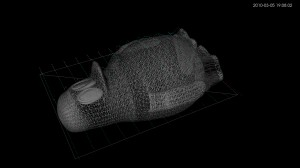I wonder if anyone is still interested in this stuff? Given enough time I would like to develop waterline-paths and an octree-based cutting simulator also. It would help if these algorithms were incorporated in a CAD-program, or someone would develop a GUI.

## OpenMP test on i7

Here's a simple piece of c-code (try zipped version) for testing how to parallelize code with OpenMP. It compiles with
`gcc -fopenmp -lm otest.c`

The CPU-load while running looks like this: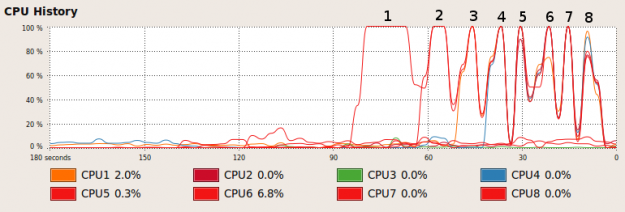Looks like two logical CPUs never get used (two low lines beyond "5" in the chart). It outputs some timing information:
``` running with 1 threads: runtime = 17.236827 s clock=17.230000 running with 2 threads: runtime = 8.624231 s clock=17.260000 running with 3 threads: runtime = 5.791805 s clock=17.090000 running with 4 threads: runtime = 5.241023 s clock=20.820000 running with 5 threads: runtime = 4.107738 s clock=20.139999 running with 6 threads: runtime = 4.045839 s clock=20.240000 running with 7 threads: runtime = 4.056122 s clock=20.280001 running with 8 threads: runtime = 4.062750 s clock=20.299999 ```
which can be plotted like this: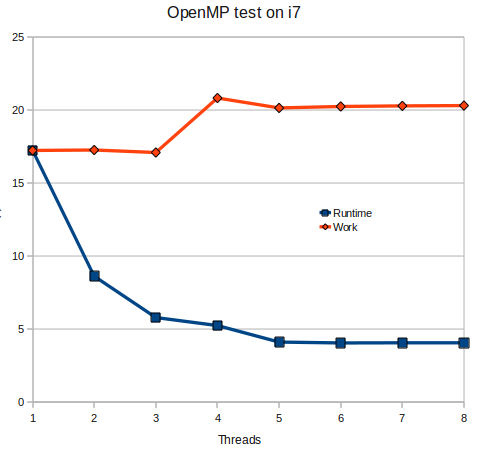I'm measuring the clock-cycles spent by the program using clock(), which I hope is some kind of measure of how much work is performed. Note how the amount of work increases due to overheads related to creating threads and communication between them. Another plot shows the speedup: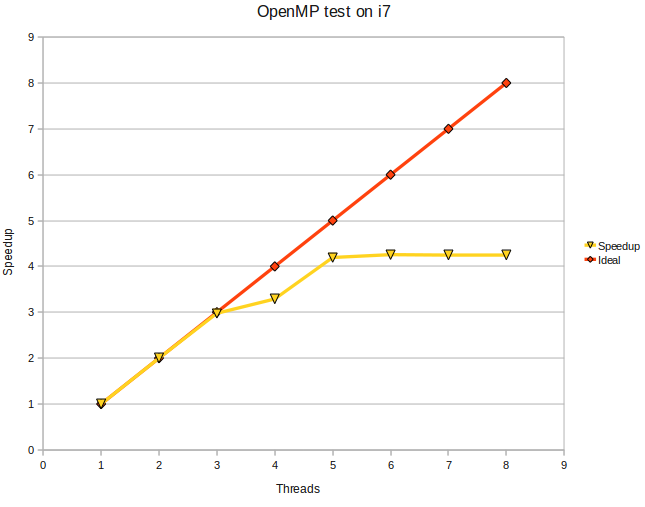The i7 uses Hyper Threading to present 8 logical CPUs to the system with only 4 physical cores. Anyone care to run this on a real 8-core machine ? 🙂

Next stop is getting this to work from a Boost Python extension.

## More pystones with shedskin

As I'm very much an amateur programmer with not too much time to learn new stuff I've decided my CAM-algorithms are going to be written in Python (don't hold your breath, they'll be online when they'll be online...). The benefits of rapid development will more than outweigh the performance issues of Python at this stage.

But then I found Mark Dufour's project shedskin (see also blog here and Mark's MSc thesis here), a Python to C++ compiler! Can you have the best of both worlds? Develop and debug your code interactively with Python and then, when you're happy with it, translate it automagically over to C++ and have it run as fast as native code?

Well, shedskin doesn't support any and all python constructs (yet?), and only a limited number of modules from the standard library are supported. But still I think it's a pretty cool tool. For someone who doesn't look forward to learning C++ from the ground up typing '`shedskin -e myprog.py`' followed by '`make`' is just a very simple way to create fast python extensions! As a test, I ran shedskin on the pystone benchmark and called both the python and c++ version from my multiprocessing test-code:

Python version

```Processes	Pystones	Wall time	pystones/s	Speedup
1		50000		0.7		76171		1.0X
2		100000		0.7		143808		1.9X
3		150000		0.7		208695		2.7X
4		200000		0.8		264410		3.5X
5		250000		1.0		244635		3.2X
6		300000		1.2		259643		3.4X
```

'shedskinned' C++ version

```Processes	Pystones	Wall time		pystones/s	Speedup
1		5000000			2.9		1696625		1.0X
2		10000000		3.1		3234625		1.9X
3		15000000		3.1		4901829		2.9X
4		20000000		3.4		5968676		3.5X
5		25000000		4.4		5714151		3.4X
6		30000000		5.1		5890737		3.5X
```

A speedup of around 20x.